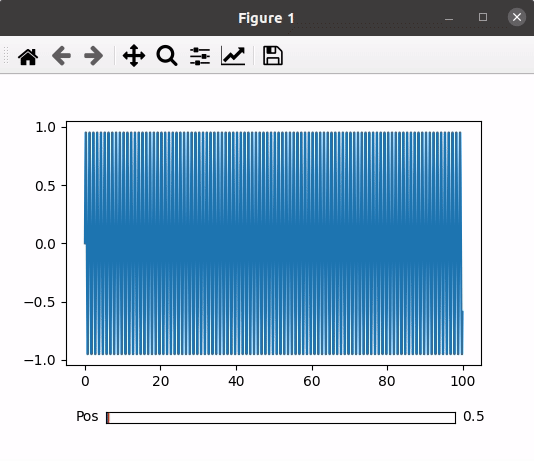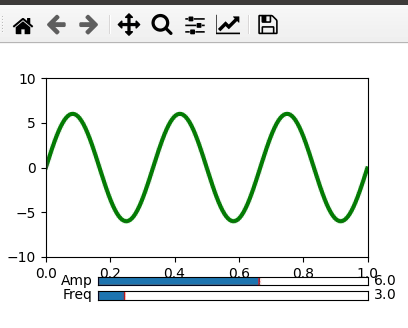Related Articles

# Python – Scroll through Plots

• Last Updated : 26 Mar, 2021

In python, we can plot graphs using many libraries and functions. Here in this article, our main focus is to add sliders to our plots. But before moving towards the slider, it is necessary to know how to plot a basic plot in python using matplotlib.pyplot.

## Scrolling through Plots

Scroll-through plots are the plots that update themselves as we change the scale of the plot using a slider. A slider can be added to a plot using matplotlib and plotly library. In this tutorial, we will learn about generating sliders using matplotlib.pyplot library.

Scroll through plots may not seem useful or necessary for each and every case but are surely a beneficial feature. There are cases where the data is huge and cannot fit into a dynamic plot and even if it fits there will be a collision between the points in the plot. Hence, using a slider will reduce collisions between the points and make it look presentable and clean. Also, it will be easy to study.

Slider() Function: Slider() function is a function that is present in matplotlib.widgets and helps the programmer to add a slider into the plots. The basic syntax of a matplotlib.widget.Slider() is

Syntax: matplotlib.widgets.Slider(axis, label, val_min, val_max, valinit=0.5, valfmt=None, closed_min=True, closed_max=True, slider_min=None, slider_max=None, dragging=True, val_step=None, orientation=’horizontal’,  **kwargs)

Example 1: Scroll through sliders.

Approach:

• Import modules
• Set the plot and axis to the subplots
• Adjust the size of the slider widget
• Set some dummy data to the x and y-axis
• Plot the graph using the dummy data
• Set the position of the slider and plot
• Define an update function that will change the graph representation when we move the slider
• Set the update function to the slider using on_changed() function to implement the changes.
• Plot the graph along with the slider.

Below is the implementation:

## Python3

 `# Import libraries using import keyword``import` `numpy as np``import` `matplotlib.pyplot as plt``from` `matplotlib.widgets ``import` `Slider`` ` `# Setting Plot and Axis variables as subplots()``# function returns tuple(fig, ax)``Plot, Axis ``=` `plt.subplots()`` ` `# Adjust the bottom size according to the``# requirement of the user``plt.subplots_adjust(bottom``=``0.25``)`` ` `# Set the x and y axis to some dummy data``t ``=` `np.arange(``0.0``, ``100.0``, ``0.1``)``s ``=` `np.sin(``2``*``np.pi``*``t)`` ` `# plot the x and y using plot function``l ``=` `plt.plot(t, s)`` ` `# Choose the Slider color``slider_color ``=` `'White'`` ` `# Set the axis and slider position in the plot``axis_position ``=` `plt.axes([``0.2``, ``0.1``, ``0.65``, ``0.03``],``                         ``facecolor ``=` `slider_color)``slider_position ``=` `Slider(axis_position,``                         ``'Pos'``, ``0.1``, ``90.0``)`` ` `# update() function to change the graph when the``# slider is in use``def` `update(val):``    ``pos ``=` `slider_position.val``    ``Axis.axis([pos, pos``+``10``, ``-``1``, ``1``])``    ``Plot.canvas.draw_idle()`` ` `# update function called using on_changed() function``slider_position.on_changed(update)`` ` `# Display the plot``plt.show()`

Output:Example 2: Scroll through multiple sliders.

Approach:

• Import modules
• Set the plot and axis to the subplots
• Adjust the size of the slider widget.
• Set amplitude to x-axis and frequency to y-axis using some dummy data.
• Plot the graph using the data and give some basic functions such as color change or size change according to the requirement.
• Set the position of amplitude slider, frequency slider, and the plot.
• Define an update function which will change the graph representation when we move any of the sliders.
• Set the update function to both the sliders by using on_changed() function.
• Plot the graph along with the sliders.

Below is the implementation:

## Python3

 `# Importing Libraries using import function``import` `numpy as np``import` `matplotlib.pyplot as plt``from` `matplotlib.widgets ``import` `Slider`` ` `# Setting Plot and Axis variables as subplots()``# function returns tuple(fig, ax)``fig, ax ``=` `plt.subplots()`` ` `# Adjust the bottom size according to the``# requirement of the user``plt.subplots_adjust(bottom ``=` `0.25``)`` ` `# Set the x and y axis to some dummy data``t ``=` `np.arange(``0.0``, ``1.0``, ``0.001``)``# Initial values of amplitude anf frequency ``# are denoted by a0 and f0``a0 ``=` `6``f0 ``=` `3``s ``=` `a0``*``np.sin(``2``*``np.pi``*``f0``*``t)`` ` `# plot the x and y using plot function``Plot, ``=` `plt.plot(t, s, lw ``=` `3``, color ``=` `'green'``)``plt.axis([``0``, ``1``, ``-``10``, ``10``])`` ` `# Choose the Slider color``axcolor ``=` `"White"`` ` `# Set the frequency and amplitude axis``frequency_axis ``=` `plt.axes([``0.25``, ``0.1``, ``0.65``, ``0.03``], ``                          ``facecolor ``=` `axcolor)``amplitude_axis ``=` `plt.axes([``0.25``, ``0.15``, ``0.65``, ``0.03``], ``                          ``facecolor ``=` `axcolor)`` ` `# Set the slider for frequency and amplitude``frequency_slider ``=` `Slider(frequency_axis, ``'Freq'``, ``                          ``0.1``, ``30.0``, valinit ``=` `f0)``amplitude_slider ``=` `Slider(amplitude_axis, ``'Amp'``, ``                          ``0.1``, ``10.0``, valinit ``=` `a0)`` ` `# update() function to change the graph when the``# slider is in use``def` `update(val):``    ``amp ``=` `amplitude_slider.val``    ``freq ``=` `frequency_slider.val``    ``Plot.set_ydata(amp``*``np.sin(``2``*``np.pi``*``freq``*``t))``     ` `# update function called using on_changed() function ``# for both frequency and amplitude``frequency_slider.on_changed(update)``amplitude_slider.on_changed(update)`` ` `# Display the plot``plt.show()`

Output:Attention geek! Strengthen your foundations with the Python Programming Foundation Course and learn the basics.

To begin with, your interview preparations Enhance your Data Structures concepts with the Python DS Course. And to begin with your Machine Learning Journey, join the Machine Learning – Basic Level Course

My Personal Notes arrow_drop_up